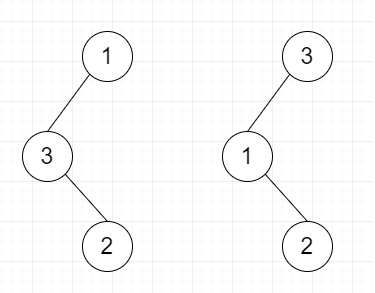# Recover Binary Search Tree in C++

C++Server Side ProgrammingProgramming

#### C in Depth: The Complete C Programming Guide for Beginners

45 Lectures 4.5 hours

#### Practical C++: Learn C++ Basics Step by Step

Most Popular

50 Lectures 4.5 hours

#### Master C and Embedded C Programming- Learn as you go

66 Lectures 5.5 hours

Suppose we have one binary search tree, now consider two elements of this BST is swapped, so we have to recover this binary search tree.

So if the given tree is like below (first one), the recovered tree will be (second one) −To solve this, we will follow these steps −

• Define some prev, first, second reference for nodes

• Define one method called findProblem(), this will take node

• if node is null, then return

• call findProblem(left of node)

• if prev is not null and value of prev > value of node, then

• if first is null, then first = prev

• second := node

• prev := node

• call findProblem(right of node)

• From the main method do the following −

• initialize prev, first and second as null, call findProblem(root) and swap values of first and second nodes

## Example (C++)

Let us see the following implementation to get a better understanding −

Live Demo

#include <bits/stdc++.h>
using namespace std;
class TreeNode{
public:
int val;
TreeNode *left, *right;
TreeNode(int data){
val = data;
left = NULL;
right = NULL;
}
};
void insert(TreeNode **root, int val){
queue<TreeNode*> q;
q.push(*root);
while(q.size()){
TreeNode *temp = q.front();
q.pop();
if(!temp->left){
if(val != NULL)
temp->left = new TreeNode(val);
else
temp->left = new TreeNode(0);
return;
} else {
q.push(temp->left);
}
if(!temp->right){
if(val != NULL)
temp->right = new TreeNode(val);
else
temp->right = new TreeNode(0);
return;
} else {
q.push(temp->right);
}
}
}
TreeNode *make_tree(vector<int> v){
TreeNode *root = new TreeNode(v);
for(int i = 1; i<v.size(); i++){
insert(&root, v[i]);
}
return root;
}
void tree_level_trav(TreeNode*root){
if (root == NULL) return;
cout << "[";
queue q;
TreeNode *curr;
q.push(root);
q.push(NULL);
while (q.size() > 1) {
curr = q.front();
q.pop();
if (curr == NULL){
q.push(NULL);
} else {
if(curr->left)
q.push(curr->left);
if(curr->right)
q.push(curr->right);
if(curr == NULL || curr->val == 0){
cout << "null" << ", ";
}else{
cout << curr->val << ", ";
}
}
}
cout << "]"<<endl;
}
class Solution {
public:
TreeNode* prev;
TreeNode* first;
TreeNode* second;
void swapValue(TreeNode* first, TreeNode* second){
int x = first->val;
first->val = second -> val;
second->val = x;
}
void findProblem(TreeNode* node){
if(!node || node->val == 0)return;
findProblem(node->left);
if((prev!=NULL && prev->val != 0) && prev->val> node->val){
if(!first){
first = prev;
}
second = node;
}
prev = node;
findProblem(node->right);
}
void recoverTree(TreeNode* root) {
prev = first = second = NULL;
findProblem(root);
swapValue(first, second);
}
};
main(){
vector<int> v = {1,3,NULL,NULL,2};
TreeNode *root = make_tree(v);
Solution ob;
ob.recoverTree(root);
tree_level_trav(root);
}

## Input

{1,3,NULL,NULL,2}

## Output

[3, 1, null, null, 2, ]